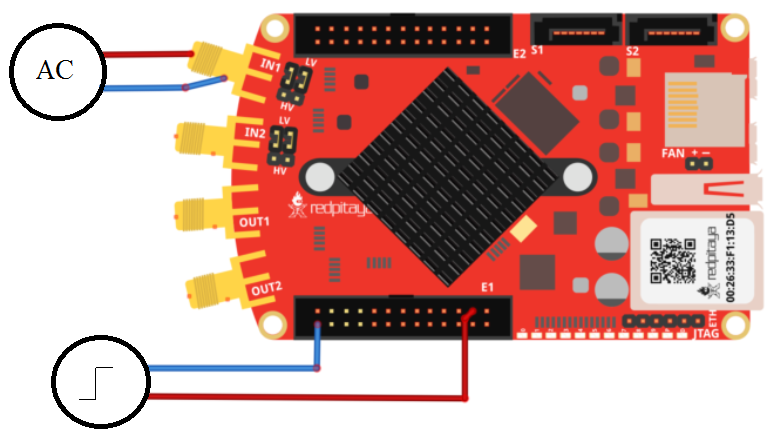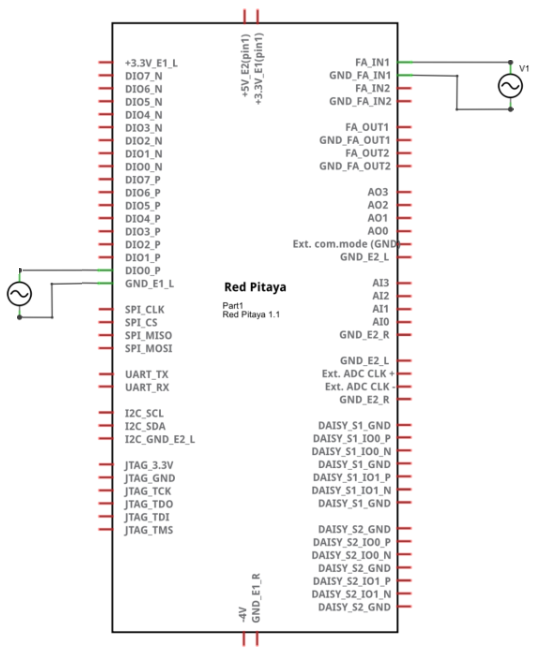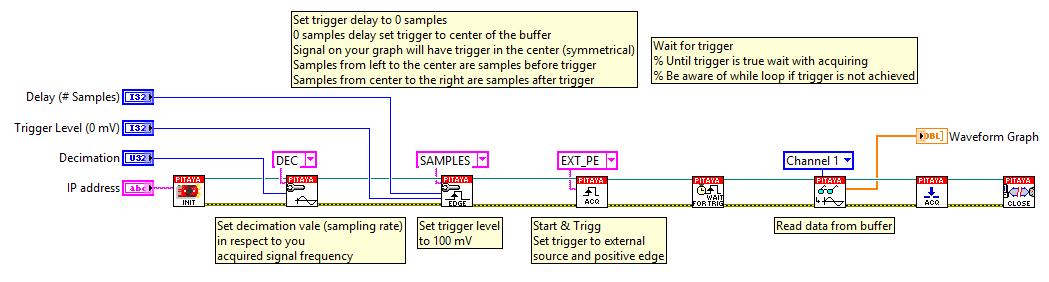# 2.3.1.4.4.2. Signal acquisition on external trigger¶

## 2.3.1.4.4.2.1. Description¶

This example shows how to acquire 16k samples of signal on fast analog inputs. Signal will be acquired when the external trigger condition is meet. Time length of the acquired signal depends on the time scale of a buffer that can be set with a decimation factor. Decimations and time scales of a buffer are given in the table. Voltage and frequency ranges depends on Red Pitaya model.

## 2.3.1.4.4.2.2. Required hardware¶

• Red Pitaya device

• Signal (function) generator

Wiring example for STEMlab 125-14 & STEMlab 125-10:## 2.3.1.4.4.2.3. Circuit¶## 2.3.1.4.4.2.4. Code - MATLAB®¶

```The code is written in MATLAB. In the code we use SCPI commands and TCP/IP communication. Copy code to MATLAB editor
and press run.

%% Define Red Pitaya as TCP/IP object
clear all
close all
clc
IP= '192.168.178.111';                % Input IP of your Red Pitaya...
port = 5000;
tcpipObj = tcpip(IP, port);
tcpipObj.InputBufferSize = 16384*32;

%% Open connection with your Red Pitaya

fopen(tcpipObj);
tcpipObj.Terminator = 'CR/LF';

flushinput(tcpipObj);
flushoutput(tcpipObj);

% Set decimation vale (sampling rate) in respect to you
% acquired signal frequency

fprintf(tcpipObj,'ACQ:RST');
fprintf(tcpipObj,'ACQ:DEC 1');
fprintf(tcpipObj,'ACQ:TRIG:LEV 0');

% Set trigger delay to 0 samples
% 0 samples delay set trigger to center of the buffer
% Signal on your graph will have trigger in the center (symmetrical)
% Samples from left to the center are samples before trigger
% Samples from center to the right are samples after trigger

fprintf(tcpipObj,'ACQ:TRIG:DLY 0');

% for SIGNALlab device there is a possiblity to set trigger threshold
% fprintf(tcpipObj,'ACQ:TRIG:EXT:LEV 1')

%% Start & Trigg
% Trigger source setting must be after ACQ:START
% Set trigger to source 1 positive edge

fprintf(tcpipObj,'ACQ:START');
% After acquisition is started some time delay is needed in order to acquire fresh samples in to buffer
% Here we have used time delay of one second but you can calculate exact value taking in to account buffer
% length and smaling rate
pause(1)

fprintf(tcpipObj,'ACQ:TRIG EXT_PE');
% Wait for trigger
% Until trigger is true wait with acquiring
% Be aware of while loop if trigger is not achieved
% Ctrl+C will stop code executing in MATLAB

while 1
trig_rsp=query(tcpipObj,'ACQ:TRIG:STAT?')

if strcmp('TD',trig_rsp(1:2))  % Read only TD

break

end
end

signal_str=query(tcpipObj,'ACQ:SOUR1:DATA?');
signal_str_2=query(tcpipObj,'ACQ:SOUR2:DATA?');

% Convert values to numbers.% First character in string is “{“
% and 2 latest are empty spaces and last is “}”.

signal_num=str2num(signal_str(1,2:length(signal_str)-3));
signal_num_2=str2num(signal_str_2(1,2:length(signal_str_2)-3));

plot(signal_num)
hold on
plot(signal_num_2,'r')
grid on
ylabel('Voltage / V')
xlabel('samples')

fclose(tcpipObj)
```

## 2.3.1.4.4.2.5. Code - Python¶

```#!/usr/bin/python

import sys
import redpitaya_scpi as scpi
import matplotlib.pyplot as plot

rp_s = scpi.scpi(sys.argv)

rp_s.tx_txt('ACQ:DEC 8')
rp_s.tx_txt('ACQ:TRIG:LEVEL 100')
rp_s.tx_txt('ACQ:START')
rp_s.tx_txt('ACQ:TRIG EXT_PE')

while 1:
rp_s.tx_txt('ACQ:TRIG:STAT?')
if rp_s.rx_txt() == 'TD':
break

rp_s.tx_txt('ACQ:SOUR1:DATA?')
buff_string = rp_s.rx_txt()
buff_string = buff_string.strip('{}\n\r').replace("  ", "").split(',')
buff = list(map(float, buff_string))

plot.plot(buff)
plot.ylabel('Voltage')
plot.show()
view rawacquire_trigger_external.py
```

## 2.3.1.4.4.2.6. Code - LabVIEW¶